Overview
Getting Started
Developer Guide
API Reference
API Structure
Geometry Primitives
Lights
Materials
Effects
Scenegraph
Geometry Nodes

Screen space effects packaged as reusable shader modules in @luma.gl/effects based on the glfx library.

This is a repackaging of shader code from Evan Wallace's glfx library.

The code and documentation is included here under MIT license.

Usage

Import brightnessContrast shader module

import {brightnessContrast} from '@luma.gl/effects';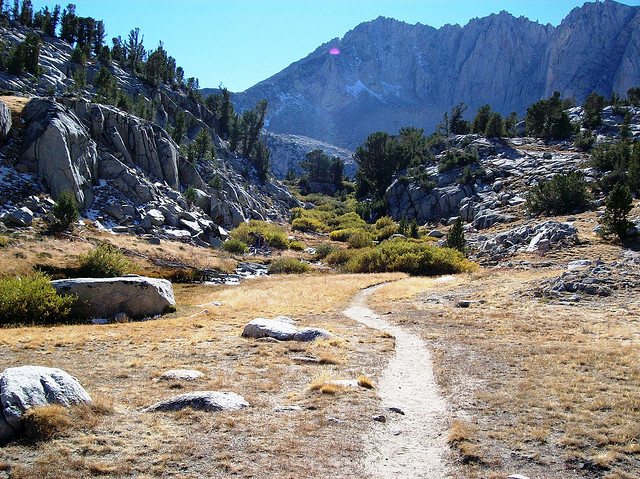Original Image

brightnessContrast

Provides additive brightness and multiplicative contrast control.

• brightness -1 to 1 (-1 is solid black, 0 is no change, and 1 is solid white). Default value is 0.
• contrast -1 to 1 (-1 is solid gray, 0 is no change, and 1 is maximum contrast). Default value is 0.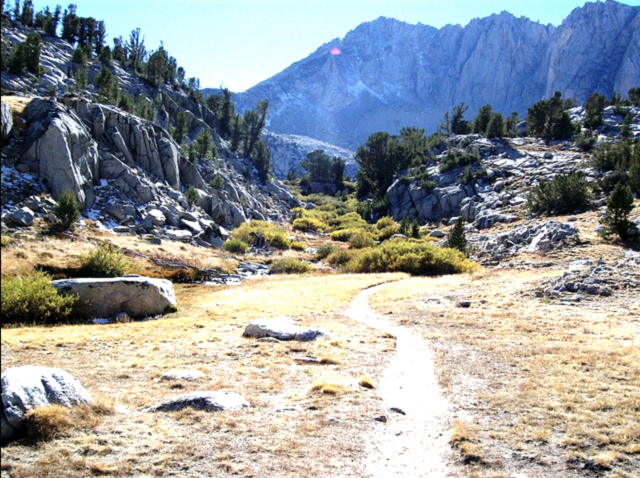Brightness / Contrast Effect

hueSaturation

Provides rotational hue and multiplicative saturation control. RGB color space can be imagined as a cube where the axes are the red, green, and blue color values.

Hue changing works by rotating the color vector around the grayscale line, which is the straight line from black (0, 0, 0) to white (1, 1, 1).

Saturation is implemented by scaling all color channel values either toward or away from the average color channel value.

• hue -1 to 1 (-1 is 180 degree rotation in the negative direction, 0 is no change, and 1 is 180 degree rotation in the positive direction). Default value is 0.
• saturation -1 to 1 (-1 is solid gray, 0 is no change, and 1 is maximum contrast). Default value is 0.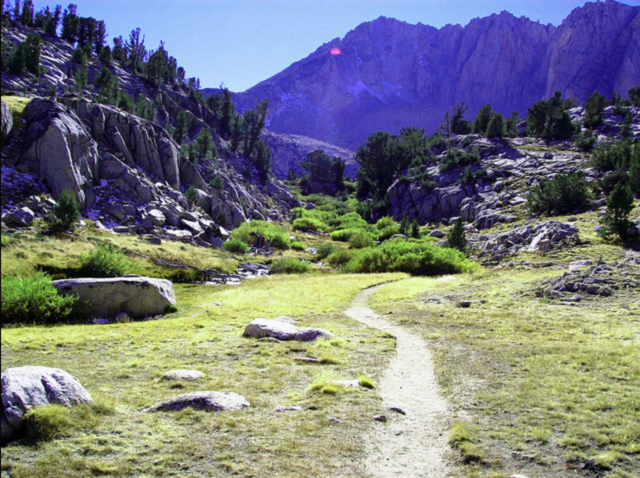Hue / Saturation Effect

noise

Adds black and white noise to the image.

• amount 0 to 1 (0 for no effect, 1 for maximum noise). Default value is 0.5.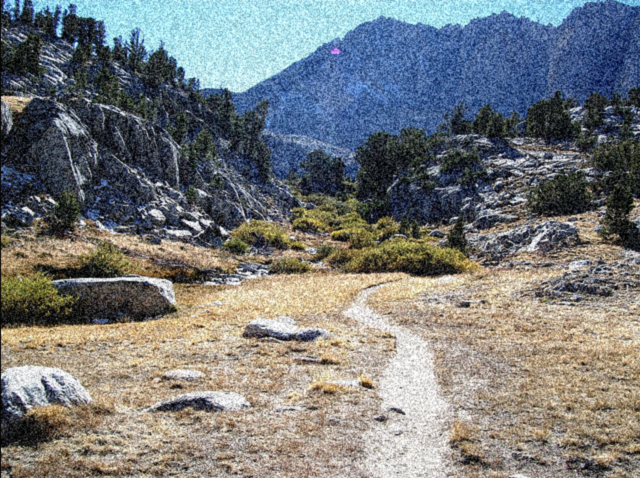Noise Effect

sepia

Gives the image a reddish-brown monochrome tint that imitates an old photograph.

• amount 0 to 1 (0 for no effect, 1 for full sepia coloring). Default value is 0.5.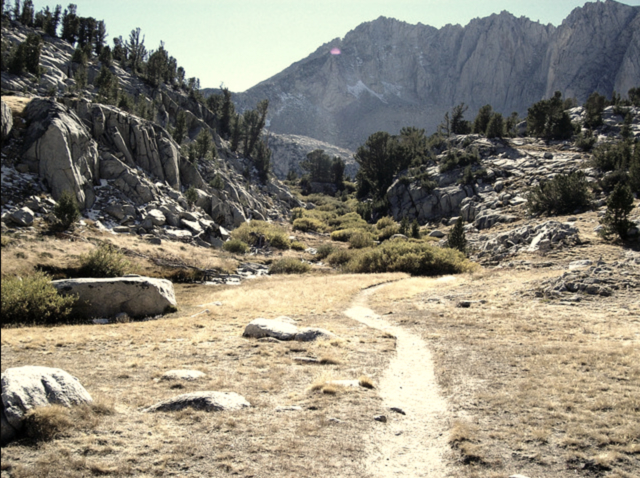Sepia Effect

vibrance

Modifies the saturation of desaturated colors, leaving saturated colors unmodified.

• amount -1 to 1 (-1 is minimum vibrance, 0 is no change, and 1 is maximum vibrance). Default value is 0.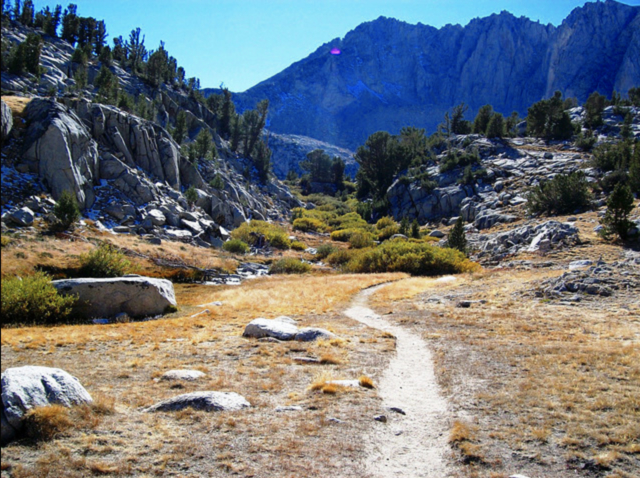Vibrance Effect

vignette

Adds a simulated lens edge darkening effect.

• size 0 to 1 (0 for center of frame, 1 for edge of frame). Default value is 0.5.
• amount 0 to 1 (0 for no effect, 1 for maximum lens darkening). Default value is 0.5.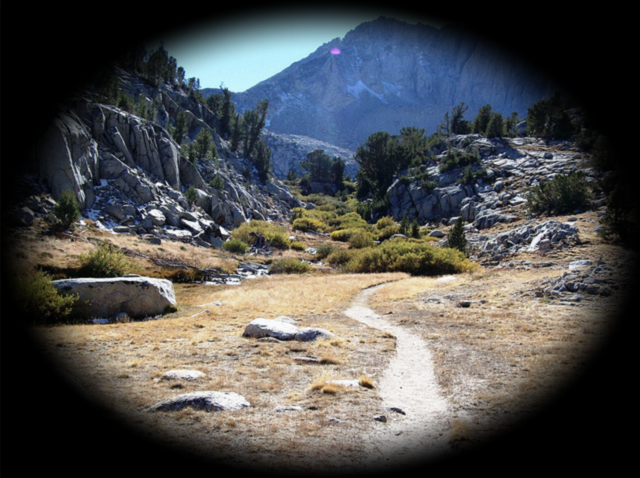Vignette Effect

tiltShift

Simulates the shallow depth of field normally encountered in close-up photography, which makes the scene seem much smaller than it actually is. This filter assumes the scene is relatively planar, in which case the part of the scene that is completely in focus can be described by a line (the intersection of the focal plane and the scene). An example of a planar scene might be looking at a road from above at a downward angle. The image is then blurred with a blur radius that starts at zero on the line and increases further from the line.

• start [x, y] coordinate of the start of the line segment. [0, 0] is the bottom left corner, [1, 1] is the up right corner. Default value is [0, 0].
• end [x, y] coordinate of the end of the line segment. [0, 0] is the bottom left corner, [1, 1] is the up right corner. Default value is [1, 1].
• blurRadius The maximum radius of the pyramid blur in pixels. Default value is 15.
• gradientRadius The distance in pixels from the line at which the maximum blur radius is reached. Default value is 200.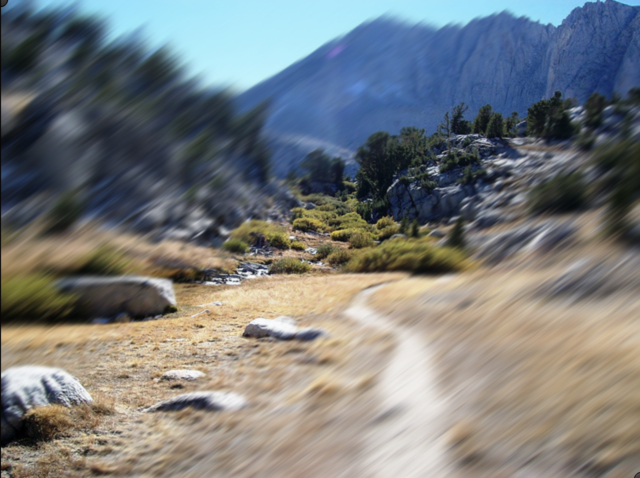Tilt Shift Effect

triangleBlur

This is the most basic blur filter, which convolves the image with a pyramid filter. The pyramid filter is separable and is applied as two perpendicular triangle filters.

• radius The radius of the pyramid in pixels convolved with the image. Default value is 20.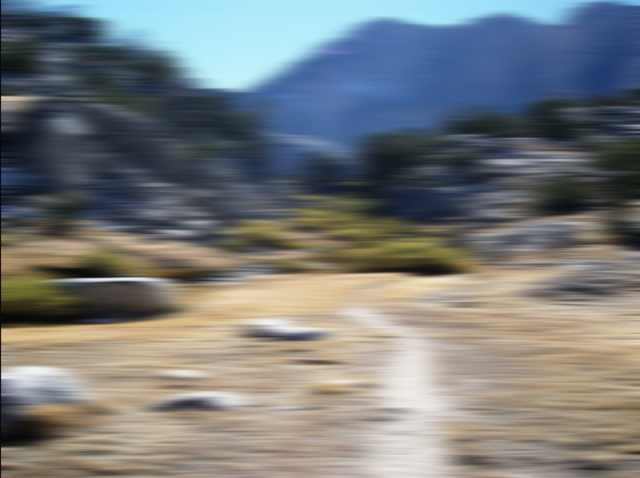Triangle Blur Effect

zoomBlur

Blurs the image away from a certain point, which looks like radial motion blur.

• center [x, y] coordinate of the blur origin. [0, 0] is the bottom left corner, [1, 1] is the up right corner. Default value is [0.5, 0.5].
• strength The strength of the blur. Values in the range 0 to 1 are usually sufficient, where 0 doesn't change the image and 1 creates a highly blurred image. Default value is 0.3.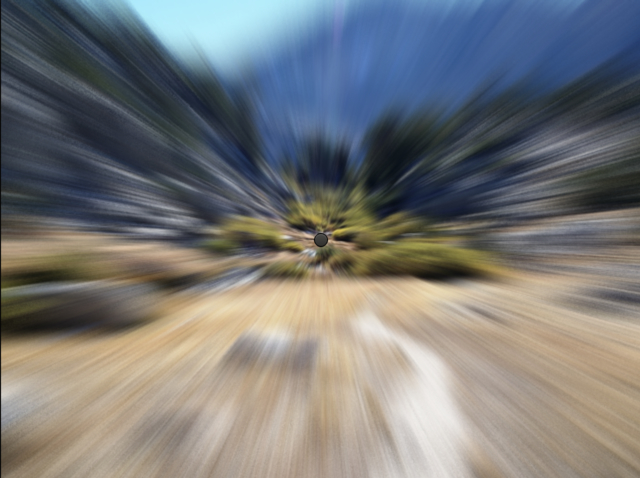Zoom Blur Effect

colorHalftone

Simulates a CMYK halftone rendering of the image by multiplying pixel values with a four rotated 2D sine wave patterns, one each for cyan, magenta, yellow, and black.

• center [x, y] coordinate of the pattern origin. [0, 0] is the bottom left corner, [1, 1] is the up right corner. Default value is [0.5, 0.5].
• angle The rotation of the pattern in radians. Default value is 1.1.
• size The diameter of a dot in pixels. Default value is 4.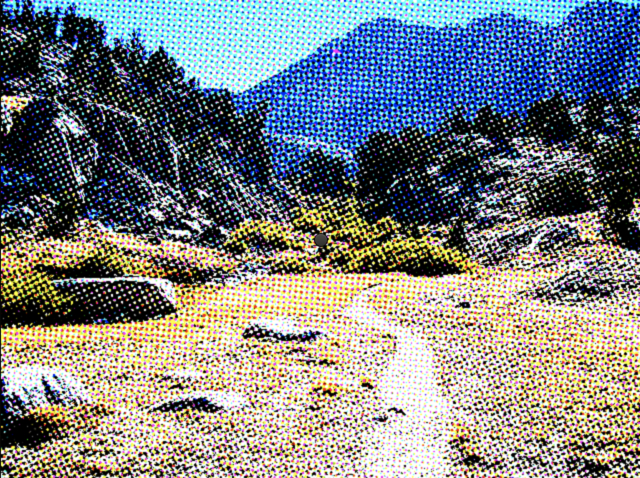Color Halftone Effect

dotScreen

Simulates a black and white halftone rendering of the image by multiplying pixel values with a rotated 2D sine wave pattern.

• center [x, y] coordinate of the pattern origin. [0, 0] is the bottom left corner, [1, 1] is the up right corner. Default value is [0.5, 0.5].
• angle The rotation of the pattern in radians. Default value is 1.1.
• size The diameter of a dot in pixels. Default value is 3.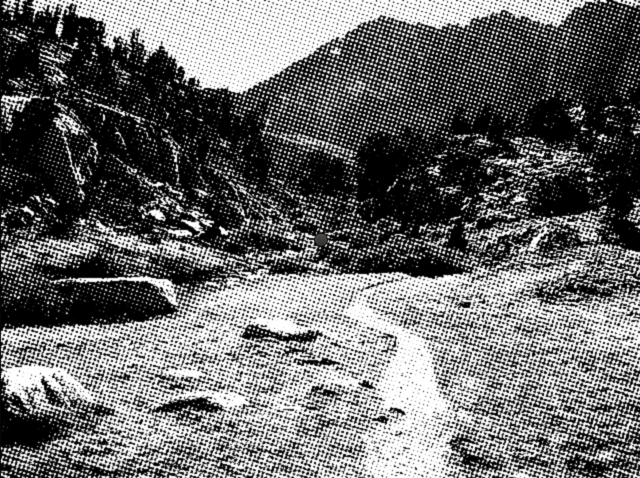Dot Screen Effect

edgeWork

Picks out different frequencies in the image by subtracting two copies of the image blurred with different radii.

• radius The radius of the effect in pixels. Default value is 2.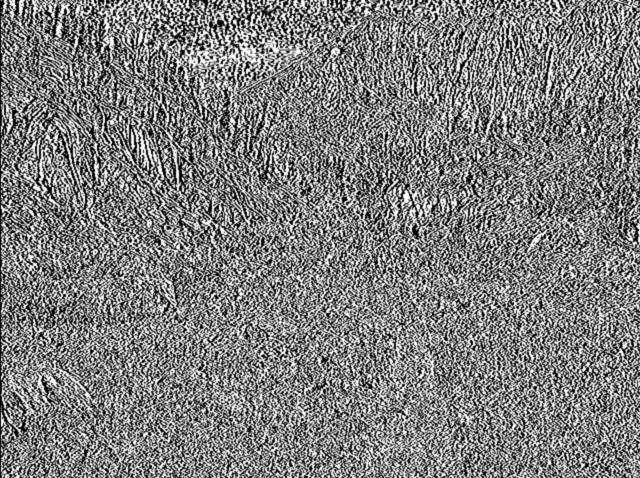Edge Work Effect

hexagonalPixelate

Renders the image using a pattern of hexagonal tiles. Tile colors are nearest-neighbor sampled from the centers of the tiles.

• center [x, y] coordinate of the pattern center. [0, 0] is the bottom left corner, [1, 1] is the up right corner. Default value is [0.5, 0.5].
• scale The width of an individual tile in pixels. Default value is 10.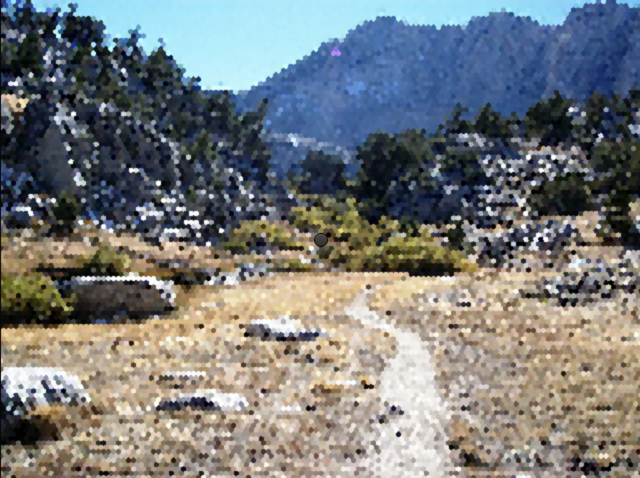Hexagonal Pixelate Effect

ink

Simulates outlining the image in ink by darkening edges stronger than a certain threshold. The edge detection value is the difference of two copies of the image, each blurred using a blur of a different radius.

• strength The multiplicative scale of the ink edges. Values in the range 0 to 1 are usually sufficient, where 0 doesn't change the image and 1 adds lots of black edges. Negative strength values will create white ink edges instead of black ones. Default value is 0.25.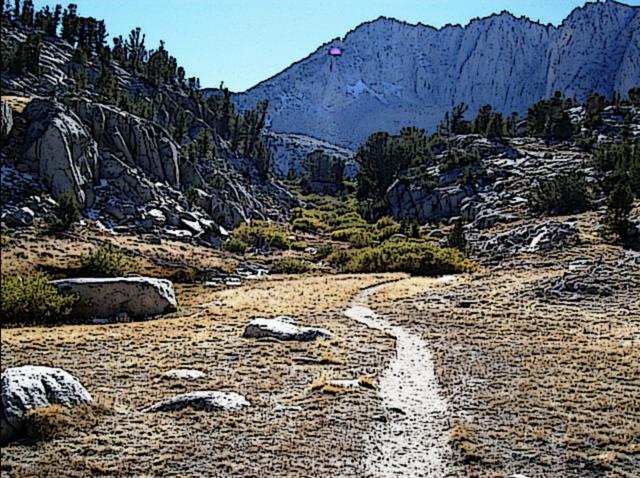Ink Effect

bulgePinch

Bulges or pinches the image in a circle.

• center [x, y] coordinate of the center of the circle of effect. [0, 0] is the bottom left corner, [1, 1] is the up right corner. Default value is [0.5, 0.5].
• radius The radius of the circle of effect in pixels. Default value is 200.
• strength -1 to 1 (-1 is strong pinch, 0 is no effect, 1 is strong bulge). Default value is 0.5.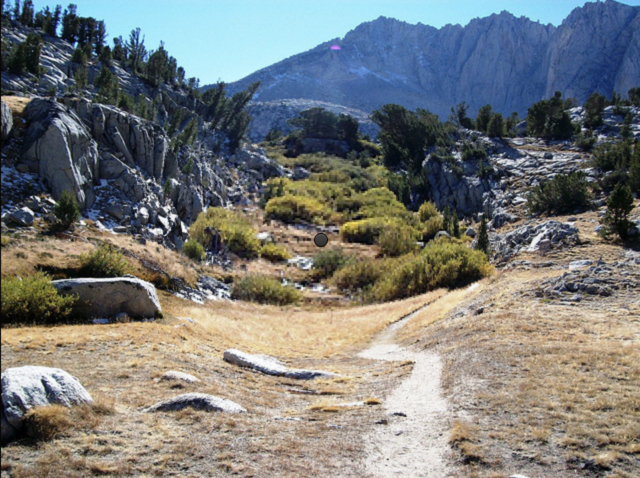Bulge Pinch Effect

swirl

Warps a circular region of the image in a swirl.

• center [x, y] coordinate of the center of the circular region. [0, 0] is the bottom left corner, [1, 1] is the up right corner. Default value is [0.5, 0.5].
• radius The radius of the circular region in pixels. Default value is 200.
• angle The angle in radians that the pixels in the center of the circular region will be rotated by. Default value is 3.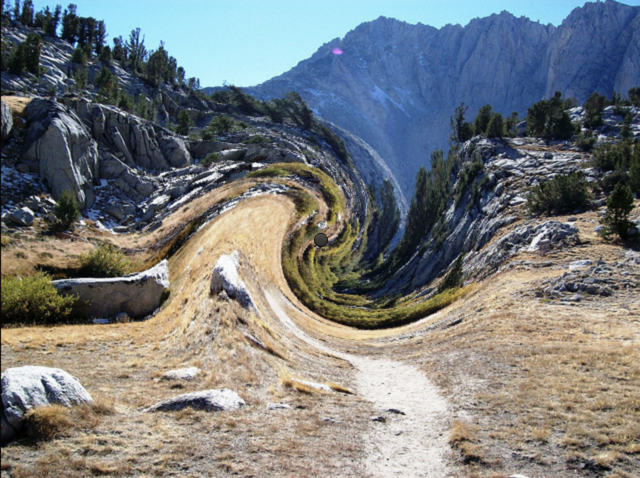Swirl Effect

Remarks

• Coordinate is based on the original image. [0, 0] is the bottom left corner, [1, 1] is the up right corner.